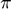Rounding Digits

Jiro's pick this week is roundsd by François Beauducel.

There are a number of entries, including this, this, this, and this, that perform this useful task of rounding numbers to specific significant digits. François provides a simple, well-implemented function with good documentation. Here's how it works.

•rounded to 3 significant digits
format long
roundsd(pi,3)
ans =
3.140000000000000
•/100 rounded to 3 significant digits:
roundsd(pi/100,3)
ans =
0.031400000000000

It works with arrays as well.

roundsd([pi pi/100],3)
ans =
3.140000000000000   0.031400000000000

Did you know that in R2014b, the round function gives you the option to do the same thing? It provides the option of rounding to significant digits or to digits respect to the decimal point.

• Round respect to the decimal point
round([pi pi/100],3)
ans =
3.142000000000000   0.031000000000000
• Round to significant digits
round([pi pi/100],3,'significant')

% Reset format
format short
ans =
3.140000000000000   0.031400000000000

Thanks, François (and others), for providing this functionality to folks using MATLAB versions prior to R2014b!